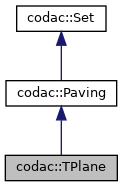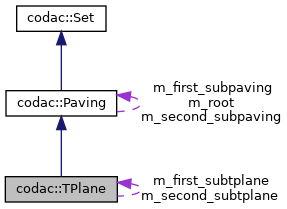codac
codac::TPlane Class Reference

Temporal representation of loops. More...

#include <codac_TPlane.h>

Inheritance diagram for codac::TPlane:[legend]
Collaboration diagram for codac::TPlane:[legend]

## Public Member Functions

TPlane (const Interval &tdomain)
Creates a 2d temporal space $[t_0,t_f]^2$. More...

void compute_loops (float precision, const TubeVector &p, const TubeVector &v)
Computes the loops (detections and proofs) as a subpaving, from the tube of positions $[\mathbf{p}](\cdot)$ and the tube of velocities $[\mathbf{v}](\cdot)$. More...

void compute_detections (float precision, const TubeVector &p)
Computes this tplane as a subpaving, from the tube of positions $[\mathbf{p}](\cdot)$ only. More...

void compute_detections (float precision, const TubeVector &p, const TubeVector &v)
Computes this tplane as a subpaving, from the tube of positions $[\mathbf{p}](\cdot)$ and the tube of velocities $[\mathbf{v}](\cdot)$. More...

void compute_proofs (const TubeVector &p)
Tries to prove the existence of loops in each detection set. More...

void compute_proofs (const TubeVector &p, const TubeVector &v)
Tries to prove the existence of loops in each detection set. More...

int nb_loops_detections () const
Returns the number of loop detections. More...

int nb_loops_proofs () const
Returns the number of proven loops. More...

const std::vector< ConnectedSubset > & detected_loops_subsets () const
Returns the set of detected loops. More...

const std::vector< IntervalVector > detected_loops () const
Returns the set of boxed detected loops. More...

const std::vector< ConnectedSubset > & proven_loops_subsets () const
Returns the set of proven loops. More...

const std::vector< IntervalVector > proven_loops () const
Returns the set of boxed proven loops. More...

Trajectory traj_loops_summary () const
Returns a temporal function of value 0 in case of no-detection, 1 in case of loop detection and 2 in case of loop proof. More...

void compute_proofs (const std::function< IntervalVector(const IntervalVector &)> &f)
Tries to prove the existence of loops in each detection set. More...Public Member Functions inherited from codac::Paving
Paving (const IntervalVector &box, SetValue value=SetValue::UNKNOWN)
Creates a paving. More...

~Paving ()
Paving destructor.

Pavingget_first_subpaving ()
Points to the first subpaving of the binary tree implementing this paving set. More...

const Pavingget_first_subpaving () const
Points to the first subpaving of the binary tree implementing this paving set. More...

Pavingget_second_subpaving ()
Points to the second subpaving of the binary tree implementing this paving set. More...

const Pavingget_second_subpaving () const
Points to the second subpaving of the binary tree implementing this paving set. More...

Pavingget_root ()
Returns a pointer to the root of the paving structure. More...

const Pavingget_root () const
Returns a pointer to the root of the paving structure. More...

Pavingget_first_leaf (SetValue val, bool without_flag=false)
Points to the first leaf of the specified value. More...

const Pavingget_first_leaf (SetValue val, bool without_flag=false) const
Points to the first leaf of the paving structure. More...

void bisect (float ratio=0.49)
Bisects this paving into two subpavings with some ratio bisection. More...

bool is_leaf () const
Returns true if this paving is made of two subpavings. More...

bool flag () const
Tests whether this paving has been flagged or not. More...

void set_flag () const
Flags this paving and all its children.

void reset_flags () const
Removes the flags of this Paving and all its children.

void get_boxes (std::list< IntervalVector > &l_subpavings, SetValue val, SetValue neg_val=SetValue::DEFAULT) const
Returns a set of boxes leaves of some value. More...

void get_pavings_intersecting (SetValue val, const IntervalVector &box_to_intersect, std::vector< const Paving *> &v_subpavings, bool no_degenerated_intersection=false) const
Returns a set of Paving leaves of some value and intersecting a given box. More...

void get_neighbours (std::vector< const Paving *> &v_neighbours, SetValue val=SetValue::IN|SetValue::OUT|SetValue::UNKNOWN, bool without_flag=false) const
Returns the neighbors (adjacent items) of this Paving, having some value. More...

std::vector< ConnectedSubsetget_connected_subsets (bool sort_by_size=false) const
Returns the set of connected subsets of this paving. More...Public Member Functions inherited from codac::Set
Set (const IntervalVector &box, SetValue value=SetValue::UNKNOWN)
Creates a set. More...

~Set ()
Set destructor.

SetValue value () const
Returns the value of this set. More...

int size () const
Returns the dimension of the paving. More...

const IntervalVector & box () const
Returns the n-dimensional box representing this set. More...

void set_value (SetValue value)
Sets the integer value for this set. More...

## Static Public Member Functions

static void verbose (bool verbose=true)
Enables verbose mode for displaying information related to loops computations. More...

## Protected Member Functions

void compute_detections (float precision, const TubeVector &p, const TubeVector &v, bool with_derivative, bool extract_subsets)
Recursive computation of the tplane, from the tube of positions $[\mathbf{p}](\cdot)$ and the tube of velocities $[\mathbf{v}](\cdot)$. More...

## Protected Attributes

float m_precision = 0.
precision of the SIVIA algorithm, used later on in traj_loops_summary()

std::vector< ConnectedSubsetm_v_detected_loops
set of loops detections

std::vector< ConnectedSubsetm_v_proven_loops
set of loops proofsProtected Attributes inherited from codac::Paving
bool m_flag
optional flag, can be used by search algorithms

Pavingm_root = nullptr
pointer to the root

Pavingm_second_subpaving = nullptr
tree structureProtected Attributes inherited from codac::Set
SetValue m_value
integer value of this set

IntervalVector m_box
box representing this set

## Detailed Description

Temporal representation of loops.

A t-plane is a 2d temporal space $[t_0,t_f]^2$ that may contain t-pairs corresponding to looped trajectories. Considering 2d trajectories $\mathbf{p}(\cdot):[t_0,t_f]\to\mathbb{R}^2$, we temporally define the set of loops as: $\mathbb{T}^*=\left\{(t_1,t_2)\in [t_0,t_f]^2 \mid \mathbf{p}(t_1)=\mathbf{p}(t_2) , t_1 < t_2 \right\}$

This class allows to detect and prove loops considering uncertainties on $\mathbf{p}(\cdot)$ or in cases where only the velocities $\mathbf{v}(\cdot)$ of the robot are at hand.

This has been the object of the publications:

Loop detection of mobile robots using interval analysis
C. Aubry, R. Desmare, L. Jaulin
Automatica, 2013

Proving the existence of loops in robot trajectories
S. Rohou, P. Franek, C. Aubry, L. Jaulin
The International Journal of Robotics Research, 2018

## ◆ TPlane()

 codac::TPlane::TPlane ( const Interval & tdomain )

Creates a 2d temporal space $[t_0,t_f]^2$.

Parameters
 tdomain temporal domain $[t_0,t_f]$

## ◆ compute_loops()

 void codac::TPlane::compute_loops ( float precision, const TubeVector & p, const TubeVector & v )

Computes the loops (detections and proofs) as a subpaving, from the tube of positions $[\mathbf{p}](\cdot)$ and the tube of velocities $[\mathbf{v}](\cdot)$.

Parameters
 precision precision $\epsilon$ of the SIVIA approximation p 2d TubeVector $[\mathbf{p}](\cdot)$ for positions v 2d TubeVector $[\mathbf{v}](\cdot)$ for velocities

## ◆ compute_detections() [1/3]

 void codac::TPlane::compute_detections ( float precision, const TubeVector & p )

Computes this tplane as a subpaving, from the tube of positions $[\mathbf{p}](\cdot)$ only.

Parameters
 precision precision $\epsilon$ of the SIVIA approximation p 2d TubeVector $[\mathbf{p}](\cdot)$ for positions

## ◆ compute_detections() [2/3]

 void codac::TPlane::compute_detections ( float precision, const TubeVector & p, const TubeVector & v )

Computes this tplane as a subpaving, from the tube of positions $[\mathbf{p}](\cdot)$ and the tube of velocities $[\mathbf{v}](\cdot)$.

Parameters
 precision precision $\epsilon$ of the SIVIA approximation p 2d TubeVector $[\mathbf{p}](\cdot)$ for positions v 2d TubeVector $[\mathbf{v}](\cdot)$ for velocities

## ◆ compute_proofs() [1/3]

 void codac::TPlane::compute_proofs ( const TubeVector & p )

Tries to prove the existence of loops in each detection set.

Note
The tplane must have been computed beforehand.
Parameters
 p 2d TubeVector $[\mathbf{p}](\cdot)$ for positions

## ◆ compute_proofs() [2/3]

 void codac::TPlane::compute_proofs ( const TubeVector & p, const TubeVector & v )

Tries to prove the existence of loops in each detection set.

Note
The tplane must have been computed beforehand.
Parameters
 p 2d TubeVector $[\mathbf{p}](\cdot)$ for positions v 2d TubeVector $[\mathbf{v}](\cdot)$ for velocities

## ◆ nb_loops_detections()

 int codac::TPlane::nb_loops_detections ( ) const

Returns the number of loop detections.

Note
The tplane must have been computed beforehand.
Returns
the number of connected subsets corresponding to feasible loops

## ◆ nb_loops_proofs()

 int codac::TPlane::nb_loops_proofs ( ) const

Returns the number of proven loops.

Note
The tplane and the proofs must have been computed beforehand.
Returns
the number of connected subsets for which at least one loop has been verified

## ◆ detected_loops_subsets()

 const std::vector& codac::TPlane::detected_loops_subsets ( ) const

Returns the set of detected loops.

Note
The tplane must have been computed beforehand.
Returns
the set of connected subsets corresponding to loop detections

## ◆ detected_loops()

 const std::vector codac::TPlane::detected_loops ( ) const

Returns the set of boxed detected loops.

Note
The tplane must have been computed beforehand.
Returns
the set of boxes $[t_1]\times[t_2]$ corresponding to loop detections

## ◆ proven_loops_subsets()

 const std::vector& codac::TPlane::proven_loops_subsets ( ) const

Returns the set of proven loops.

Note
The tplane and the proofs must have been computed beforehand.
Returns
the set of connected subsets corresponding to loops proofs

## ◆ proven_loops()

 const std::vector codac::TPlane::proven_loops ( ) const

Returns the set of boxed proven loops.

Note
The tplane and the proofs must have been computed beforehand.
Returns
the set of boxes $[t_1]\times[t_2]$ corresponding to loops proofs

## ◆ traj_loops_summary()

 Trajectory codac::TPlane::traj_loops_summary ( ) const

Returns a temporal function of value 0 in case of no-detection, 1 in case of loop detection and 2 in case of loop proof.

This method is useful when used together with colormaps for display purposes.

Returns
the corresponding temporal function (trajectory)

## ◆ verbose()

 static void codac::TPlane::verbose ( bool verbose = true )
static

Enables verbose mode for displaying information related to loops computations.

Parameters
 verbose boolean

## ◆ compute_proofs() [3/3]

 void codac::TPlane::compute_proofs ( const std::function< IntervalVector(const IntervalVector &)> & f )

Tries to prove the existence of loops in each detection set.

Note
The tplane must have been computed beforehand.
Parameters
 f the inclusion function $[\mathbf{f}]:\mathbb{IR}^2\to\mathbb{IR}^2$

## ◆ compute_detections() [3/3]

 void codac::TPlane::compute_detections ( float precision, const TubeVector & p, const TubeVector & v, bool with_derivative, bool extract_subsets )
protected

Recursive computation of the tplane, from the tube of positions $[\mathbf{p}](\cdot)$ and the tube of velocities $[\mathbf{v}](\cdot)$.

Note
This method is used as recursive algorithm.
Parameters
 precision precision $\epsilon$ of the SIVIA approximation p 2d TubeVector $[\mathbf{p}](\cdot)$ for positions v 2d TubeVector $[\mathbf{v}](\cdot)$ for velocities with_derivative if true, the loop detection is made with derivative tubes given in arguments extract_subsets if true, a set of ConnectedSubset objects will be computed from this Paving

The documentation for this class was generated from the following file: